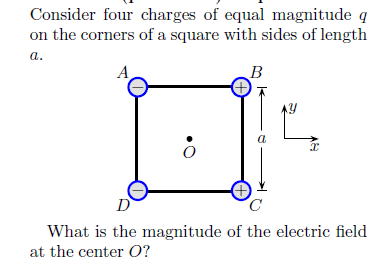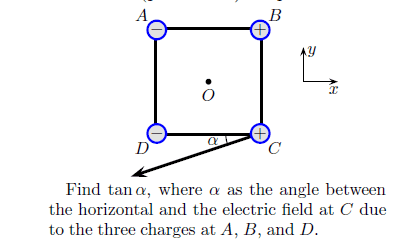# Electric Field in a Square Problem

Kirasagi

## Homework Statement

I think it would be better if I put the picture.Find the direction of the electric field.
Find the magnitude of electric field due at C due to charges A, B, and D.## Homework Equations

Pythagorean Theorem.
E = kq/r^2

## The Attempt at a Solution

For the first problem, I drew vector diagrams based on the charges at the center of the square. I ended up having 4 arrows:
O -> A (electron attraction)
O -> D (electron attraction)
O -> A (proton repulsion of charge C)
O -> D (proton repulsion of charge D)
So basically I had 2 visual arrows of pointing NW and SW from the origin. This makes the direction of the electric field to be West. Thats basically the part I only got.
For the magnitude of the electric field I used E = kq/r^2 . I know r = [√(2)/2]a. Now I got lost on what to really do (I tried a bunch of stuff but it didn't really make sense to me). The final answer for the first question is 4√2 kq/a^2

Homework Helper
Gold Member
Do you know how to sum vectors? eg Work out the x and y component of each vector. Sum all the x components and sum all the y components etc

To further simplify the equations it might help to remember that:

This is a square so 45 degree angles are involved.
cos(45)=sin(45)=0.5*SQRT(2)
Pythagorous involves squares and square roots

sorry if you know all that.

Kirasagi

All along I was doing my math wrong on adding the electric field vectors.

As r = (sqrt2/2)a

e = kq/(r^2)
e = kq/(a^2/2)
e = 2kq/(a^2) for each vector on one side.

Since theres 2 vectors on each side then it equals to 4kq/(a^2)

Combining the vectors using parallelogram rule I get 4sqrt2 kq/(a^2)

For the third question (Find the magnitude of electric field due at C due to charges A, B, and D):
Do I just solve this problem similar to the 1st question? (draw vectors based on the center O). I drew the vectors due to C and heres what I came up with:

C -> A
C -> D
B -> down

Not sure if I'm starting this correctly. Do I just add the electric field vectors again based on the superposition rule? If so how would I do the vector pointing north from B (not sure what I would put for as r).

Last edited: